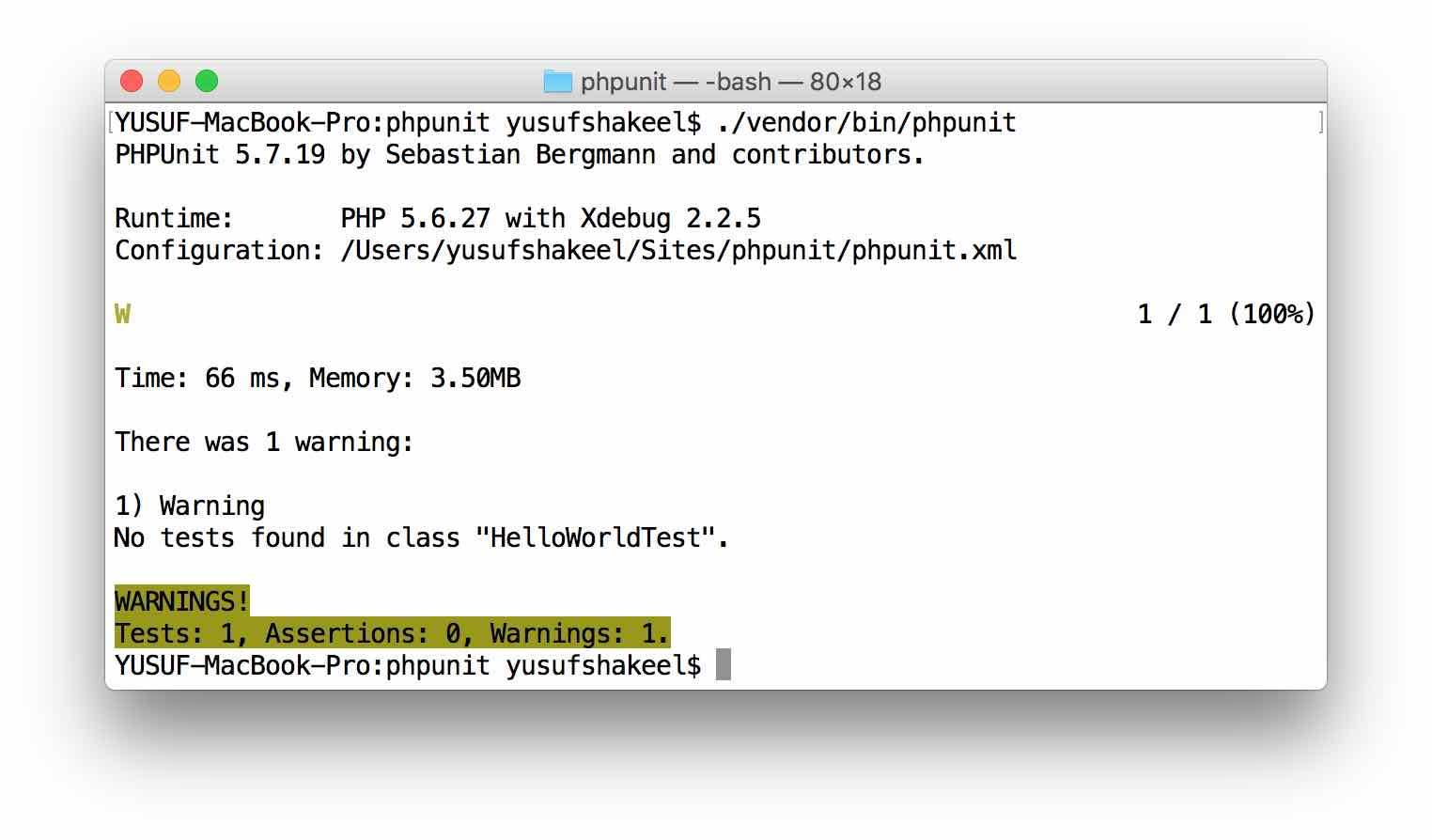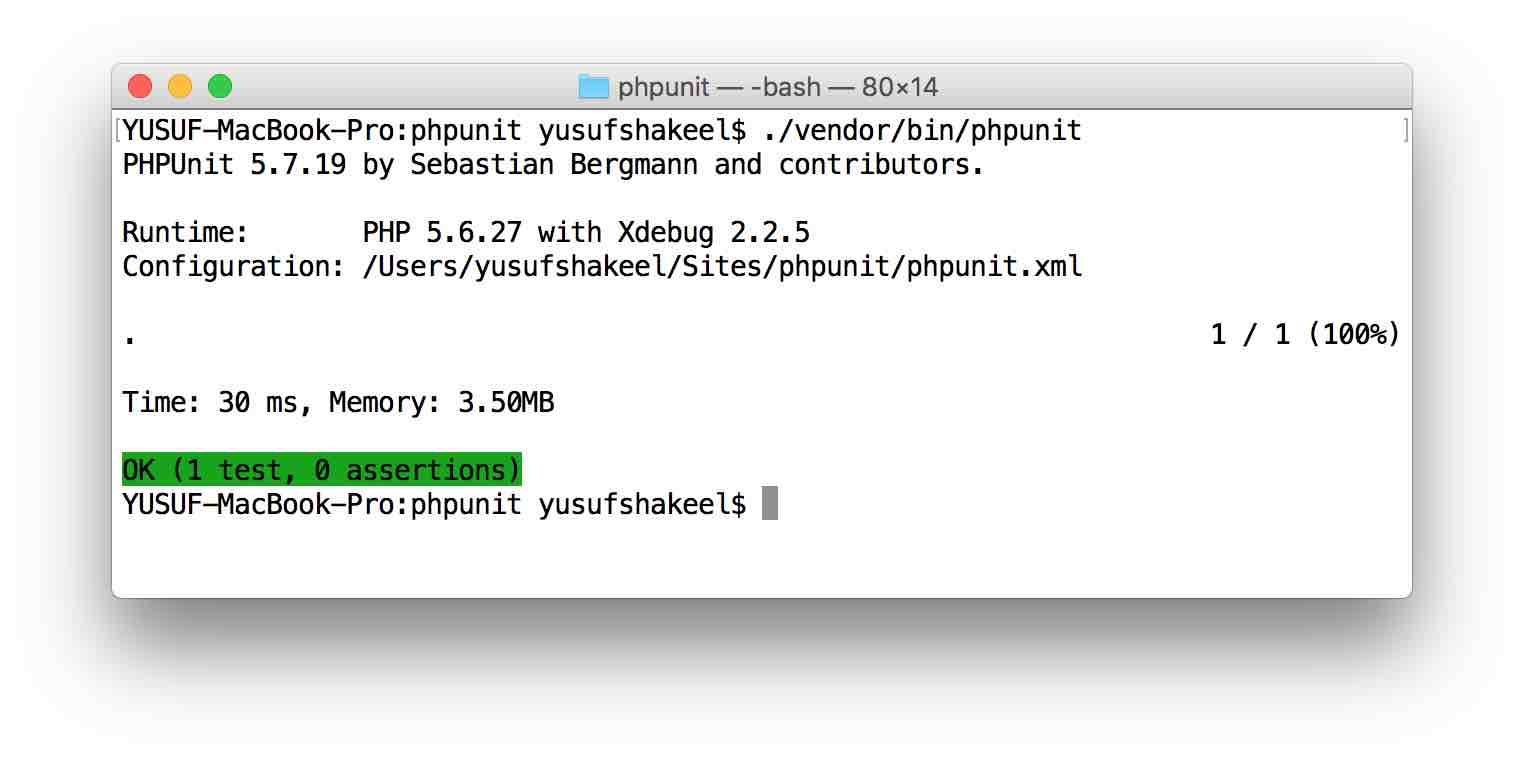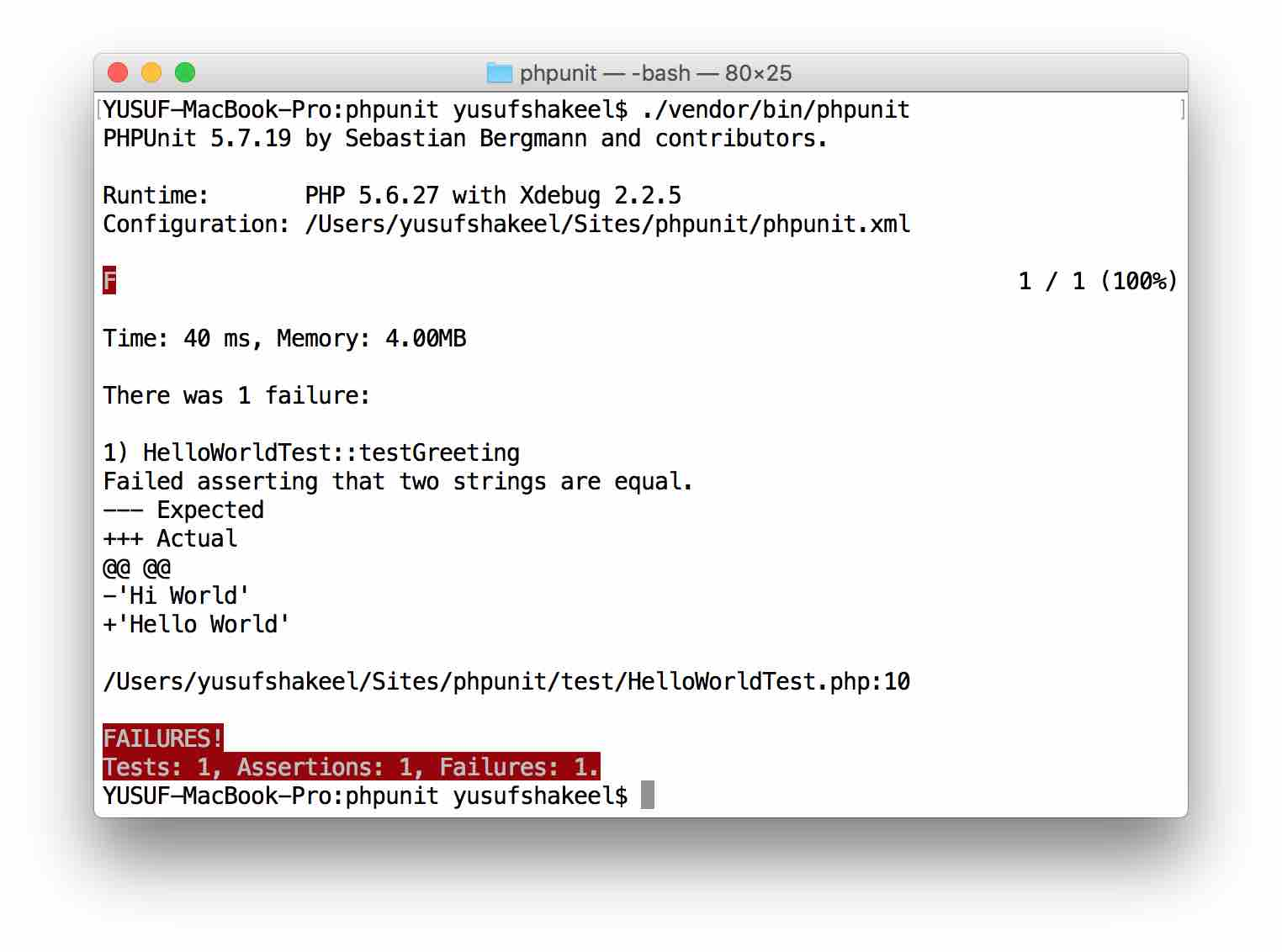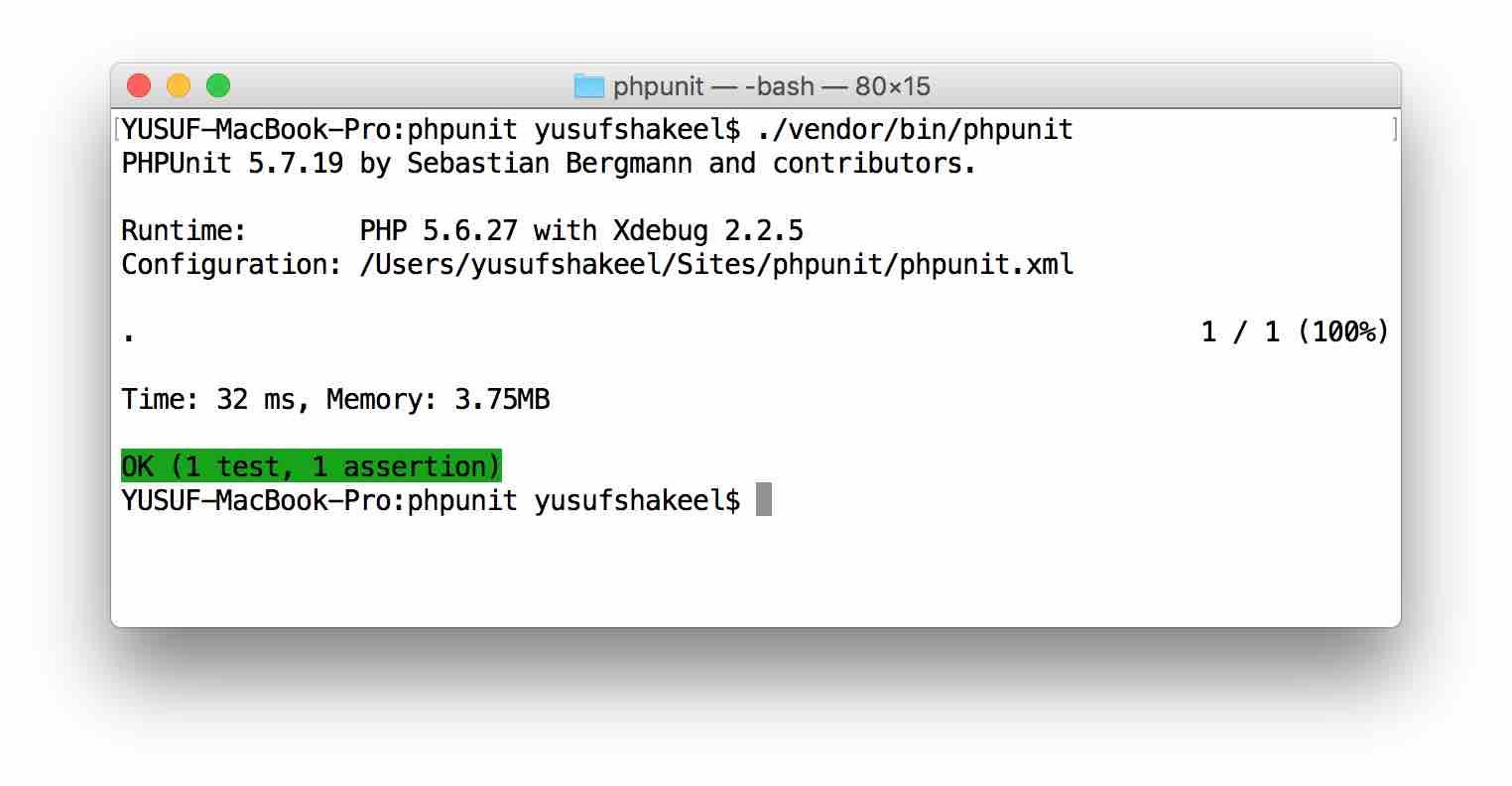# PHPUnit - Hello World Test

PHPUnitIn this tutorial we will write our first PHPUnit test.

## Hello World Test

Inside the test folder that we created in our getting started tutorial, we will go ahead and create HelloWorldTest.php file.

Write the following code inside the file.

``````<?php

class HelloWorldTest extends \PHPUnit_Framework_TestCase {

}

?>
``````

We have created a class `HelloWorldTest` and it is extending `PHPUnit_Framework_TestCase` class.

At this moment the class is empty so if we run phpunit we will get the following warning stating that there is no test in the class.

``````WARNINGS!
Tests: 1, Assertions: 0, Warnings: 1.
``````Now let us go ahead and create our first test. So, inside the class create the following method.

``````public function testGreeting() {
//some code here...
}
``````

So, we have created our first test method and named it testGreeting

It is a naming convention to add the word test before test methods.

At this moment the method is empty so if we run the phpunit again we will get the following output.

``````OK (1 test, 0 assertions)
``````This means we have 1 test but we have 0 assetions in our test. So, let us go ahead and add some more code to our `testGreeting()` method and do some assertion.

Lets say, we want to check if the value of a variable is equal to a required value.

So, we have a `\$greeting` variable in the following code and it holds the `"Hi World"` string.

Now, lets say we want to check if it is equal to the `\$requiredGreeting` value.

To perform the equals assertion we use the `assertEquals()` method.

``````public function testGreeting() {

\$greeting = "Hi World";
\$requiredGreeting = "Hello World";

\$this->assertEquals(\$greeting, \$requiredGreeting);

}
``````

If you look at the above code you can see that the required greeting message is not equal to the greeting message. So, if we run the test we will get failed result.

``````FAILURES!
Tests: 1, Assertions: 1, Failures: 1.
``````So, the above output says that "Hello World" was expected but "Hi World" was provided.

To fix this issue we have to set the value of the `\$greeting` variable to `"Hello World"`.

## Final Code

``````<?php

class HelloWorldTest extends \PHPUnit_Framework_TestCase {

public function testGreeting() {

\$greeting = "Hello World";
\$requiredGreeting = "Hello World";

\$this->assertEquals(\$greeting, \$requiredGreeting);

}

}

?>
``````

Now, if we run the test we will get the following output.

``````OK (1 test, 1 assertion)
``````And, we have success!